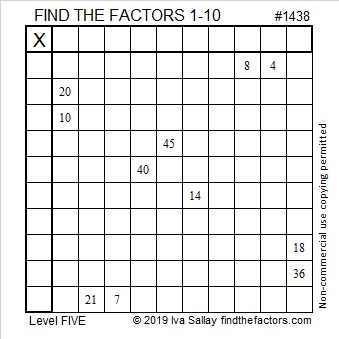# 1438 and Level 5

You can solve this puzzle by using logic and multiplication/division facts. The unique solution requires all the numbers from 1 to 10 in both the first column and the top row. Can you solve it?Print the puzzles or type the solution in this excel file:  10 Factors 1432-1442

Today’s puzzle was given the number 1438. Here are a few facts about that number:

• 1438 is a composite number.
• Prime factorization: 1438 = 2 × 719
• 1438 has no exponents greater than 1 in its prime factorization, so √1438 cannot be simplified.
• The exponents in the prime factorization are 1, and 1. Adding one to each exponent and multiplying we get (1 + 1)(1 + 1) = 2 × 2 = 4. Therefore 1438 has exactly 4 factors.
• The factors of 1438 are outlined with their factor pair partners in the graphic below.1438 is the sum of four consecutive numbers:
358  + 359 + 360 + 361 = 1438